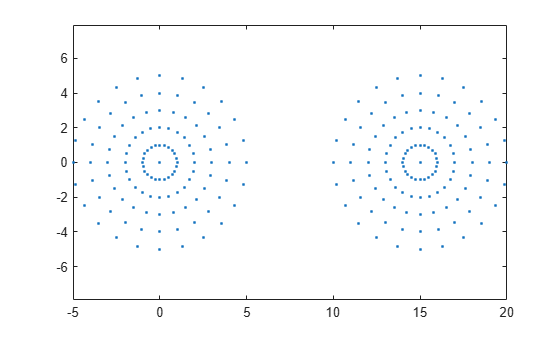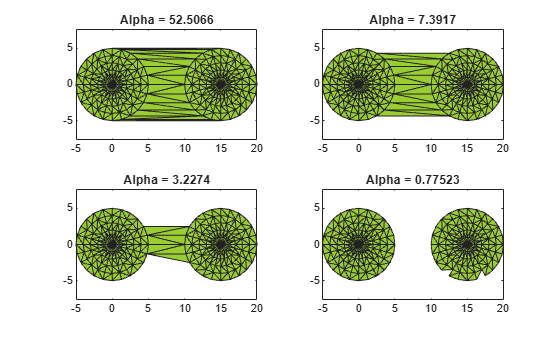# alphaSpectrum

Alpha values giving distinct alpha shapes

## Syntax

``a = alphaSpectrum(shp)``

## Description

example

````a = alphaSpectrum(shp)` returns the values of the alpha radius that produce distinct alpha shapes. `a` is in descending sorted order. Each element in `a` represents a value of the alpha radius that results in a distinct shape. The length of `a` is equal to the number of unique shapes. Values of alpha that lie between the values in `a` do not produce unique alpha shapes.```

## Examples

collapse all

Create and plot a set of 2-D points.

```th = (pi/12:pi/12:2*pi)'; x1 = [reshape(cos(th)*(1:5), numel(cos(th)*(1:5)),1); 0]; y1 = [reshape(sin(th)*(1:5), numel(sin(th)*(1:5)),1); 0]; x = [x1; x1+15;]; y = [y1; y1]; plot(x,y,'.') axis equal```Create an alpha shape for the point cloud using the default alpha radius.

`shp = alphaShape(x,y);`

Find the spectrum of critical alpha values that produce unique alpha shapes for the point cloud.

`alphaspec = alphaSpectrum(shp);`

Plot four of the unique alpha shapes generated by alpha values in the spectrum.

```for k = 1:4 alpha = alphaspec(8*k-7); shp.Alpha = alpha; subplot(2,2,k) plot(shp) title("Alpha = "+alpha) end```## Input Arguments

collapse all

Alpha shape, specified as an `alphaShape` object. For more information, see `alphaShape`.

Example: `shp = alphaShape(x,y)` creates a 2-D `alphaShape` object from the `(x,y)` point coordinates.

## Output Arguments

collapse all

Alpha values for distinct alpha shapes, returned as a column vector in descending sorted order.

## Version History

Introduced in R2014b